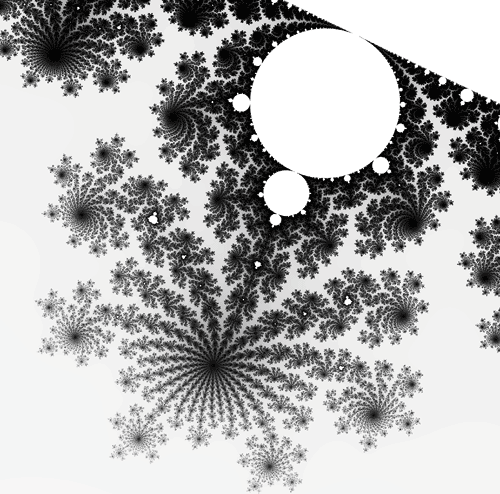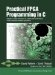# Section 13.1. Design Overview

### 13.1. Design Overview

Simply stated, fractals are infinitely complex objects with dimensions that are expressed as complex numbers. These types of objects were discovered by mathematicians around 1870, but it wasn't until Benoit Mandelbrot found (in the mid-1970s) a common mathematical relationship between the fractal objects discovered to date that the field of fractal geometry was born. One of Mandelbrot's most important contributions was to discover that fractal geometry could describe many real-world objects (which consist of both order and chaos) far better than could traditional mathematical models. Mandelbrot found a way to describe many of the structures and phenomena found in nature as an infinite set of fractal models. Such models now form the basis of weather forecasting as well as providing a basis for more mundane uses such as data compression.

Fractals are generated by performing repeated calculations on complex numbers (those with both real and imaginary components). The same formulae are repeated over and over (perhaps thousands or millions of times), with the results of one step being fed back into the next step.

#### The Mandelbrot Set

The Mandelbrot set is the best-known fractal object and was first described by Benoit Mandelbrot in 1977. This object is created by iterating a simple formula over an X-Y plane of complex numbers. For each complex number C in the plane, the algorithm iterates on the following basic calculation:

Z0 = 0

Zn+1 = Zn2 + C

This formula iteratively takes the value Zn, squares it, and adds the value of C (from an X-Y plane of complex values) to arrive at a new value for Zn+1. This point is again squared, C is added, and so on for some maximum number of iterations or until Z diverges toward infinity, whichever comes first.

The key to fractals is that the value of Z (through the course of these many iterative calculations) demonstrates dramatically different behavior depending on the value of C, which is of course a complex number. If the iteration formula is applied to every point on the X-Y plane and colored according to the behavior of Z (one color, such as white, if it diverges to infinity, and another color, such as black, if it converges), you obtain a graph such as that shown in Figure 13-1.

##### Figure 13-1. A subregion of the Mandelbrot set.This is a plot of the Mandelbrot set for a specific subregion. As this plot demonstrates, by zooming in to specific regions at the edges of the fractal objects that appear, we can find fascinating patterns. These patterns represent an infinite number of variations on the same basic theme and are similar to many of the complex (and yet seemingly random) patterns found in nature.

#### Accuracy Versus Processing Requirements

The image shown in Figure 13-1 was generated using double-precision floating-point calculations and a maximum number of iterations equal to 2000. This is the number of iterations at which we have decidedalthough not proventhat a given point will not diverge to infinity. Although a maximum iteration value of 2000 is adequate for drawing reasonably attractive pictures of fractal objects, it is probably insufficient for generating a highquality result. This is because many of the points in a given X-Y plane diverge only after many more iterations have been performed. For a high level of accuracy, in fact, we may want to have the maximum iteration value set substantially higher, perhaps even in the millions.

Why such a high iteration value? While most points in a given plane (which in Figure 13-1 range from a value of negative .3965625 to negative .380470703125 in the X axis and positive .588052734375 to positive .604144531250 in the Y axis) diverge rather quickly, other points may not diverge until a very large number of iterations have been completed. This fact becomes more apparent as the image is magnified. If the number of iterations is not sufficiently high, the edges of the diagram will not be clearly defined, and some points appear (incorrectly) to converge, perhaps showing up as black or white points of "noise" in the generated image.

The higher the iteration count, the higher the accuracy of the fractal image. From this fact it should be obvious that generating high-quality fractal images is computationally expensive. The more detail you want to observe (and the more interesting the region of the plane you are examining), the more calculations that are required. This means that if the fractal image is generated just one pixel at a time in a serial fashion on a traditional processor (such as a PC), a high-quality image may require many hoursor potentially many daysto complete.Practical FPGA Programming in C
ISBN: 0131543180
EAN: 2147483647
Year: 2005
Pages: 208

Similar book on Amazon